## Fitness F

As pointed out above is the concrete definition of the fitness function as such not part of GA. The minimal requirement is to give some value at all, bu not how. In the simple example used here Goldberg  interpreted the binary strings as encodings of decimal numbers according to the formula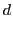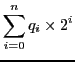(4.7)

laying the ground for the simple fitness function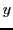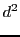(4.8)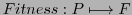The individual fitness is computed and inserted in the 7 th column of the matrix representing the population. Based on this the overall fitness is computed, the maximal fitness of all values as well as the overall average fitness. At the 8 th column the relative fitness of each member is computed.

-->for j=1:n, v=POP(j,:),  [POP(j,l+1)]= vec2dec(v,l), end

POP  =

0.    1.    1.    0.    1.    13.    169.    0.    0.    0.
1.    1.    0.    0.    0.    24.    576.    0.    0.    0.
0.    1.    0.    0.    0.    8.     64.     0.    0.    0.
1.    0.    0.    1.    1.    19.    361.    0.    0.    0.

-->[FITNESS_ALL]=fitness(POP,l)
FITNESS_ALL  =

1170.

--> cyc=1,  FITNESS_ALLLOG(cyc)=FITNESS_ALL
cyc  =

1.
FITNESS_ALLLOG  =

1170.

--> [MFITNESS]=maxfitness(POP,l)
MFITNESS  =

576.

-->  [POP,AFITNESS]=rfitness(POP,l,FITNESS_ALL)
AFITNESS  =

292.5
POP  =

0.    1.    1.    0.    1.    13.    169.    0.1444444    0.    0.
1.    1.    0.    0.    0.    24.    576.    0.4923077    0.    0.
0.    1.    0.    0.    0.    8.     64.     0.0547009    0.    0.
1.    0.    0.    1.    1.    19.    361.    0.3085470    0.    0.


Gerd Doeben-Henisch 2013-01-14